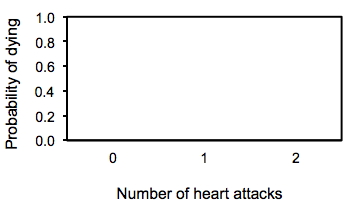## Information

For presenting scientific data in graph form, the choice is almost always scatter plots vs. bar graphs. For scientific data, any other graph style is not useful in most cases. Use either scatter plots or bar graphs for scientific data and avoid all other types.

To decide whether to choose either a scatter plot or a bar graph for your data, look at the X variable data being plotted. The X variable data determines which graph type to choose.

## Scatter Plots

Scatter plots are used when there is a real or implied continuity to the X variable data.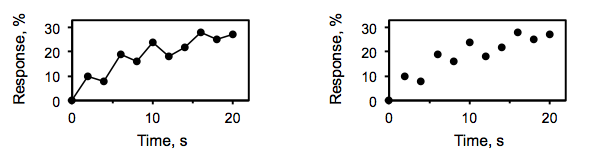Figure 2-2. Scatter plots.

You can choose to connect your data symbols with lines in a scatter plot or not, but the X-axis values have to form a continuum.

That is to say, in scatter plots the X values being graphed usually form a continuous series, like time. Each value on the X axis is connected to the ones before and after it.

## Bar Graphs

Bar graphs are used when there is no continuity between X variable data values.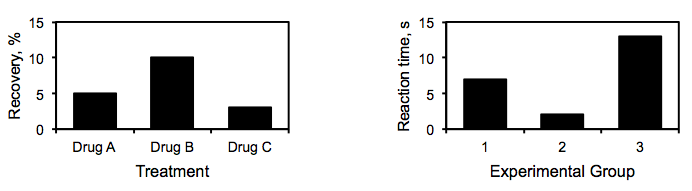Figure 2-3. Bar graphs.

You can have numbers for X variable data sets, although many times you do not, but if those numbers do not represent a continuum of values, use a bar graph.

That is to say, in bar graphs the X values being plotted aren’t really connected to each other. They are discrete separate categories.

### Lab 2 Exercises 2.1

For data that would be plotted in the following graphs, should you use a scatter plot or a bar graph? Use the axis titles to determine what is being graphed.

1. Scatter or bar? _____________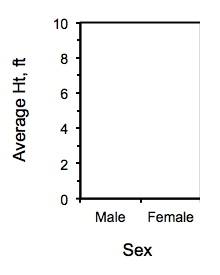2. Scatter or bar? _____________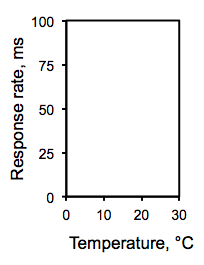3. Scatter or bar? _____________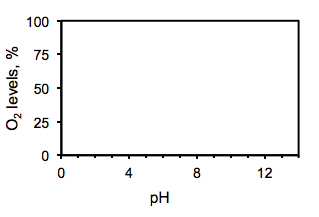4. Scatter or bar? _____________